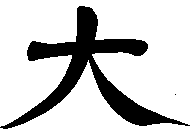In mathematical notation you will find Arabic numbers, Greek letters, German characters and other kinds of dots and strokes.

How come there is no Chinese character in math?

### Why a new formula symbol?

What is the chinese formula symbol 大 good for. What is that kind of mathematical operation, that needs a new symbol to be introduced into mathematical notation. The purpose of the operator 大 is to change the value of variables. It is the mathematical equivalent to an assignment in programming languages, which is the most elementary operation of all computational steps. It is supported by all computer algebra systems and it seems to be most intuitive to change the value of variables during a computation. Strangely enough the operation does not yet have a proper mathematical operator.

### Mathematical definition

The symbol 大 can be used to denote a value assignment operator. Above the operator we place the variable to which a new value should be assigned. Below we write the new value. The whole operator with its two arguments atop and below is applied to a formula, which we want to evaluate with the newly assigned parameters. This is mathematically precise and intuitive at the same time.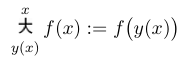Prevailing mathematical notation knows this operation as the bar operator. Its symbol is a vertical line with the value assignment in the lines index position. Other than all other mathematical operators, the bar operator is applied from the right hand side, which makes it difficult to integrate in larger formulas. Furthermore a mathematician is required states an equality in the operators index position, where by nature no equality holds.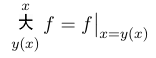### Examples

The following example demonstrates the use of the 大 operator in an operator sequence. Read from left to right we can interpret it as assigning 8 to x, then multiplying x with 5 and finaly evaluating x + 2. Alternatively, we can stick to the operators definition and evaluate from right to left.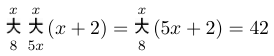Operator theory allows operators to be taken to the power of any integer number. The result is a repeated application of that operator. Computer programmers know this construction as loops with deterministic length.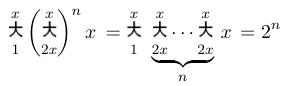## Subdirectories

View
[xml]
[htm]
[dir]
 One level up doc 2.8M 96 files Documentation logo 44K 8 files projects 1.4M 10 files sound 392K 4 files src 2.3M 279 files Source code talks 1.5M 13 files Presentations
Created on 2006-Mar-05
Stefan Dirnstorfer